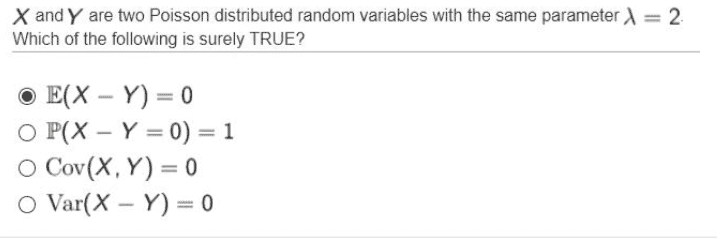# Two Poisson distributed random variables

• DottZakapa
In summary, the difference of two Poisson variables is a Skellam distribution (as opposed to a sum of Poisson variables, which is just another Poisson variable with parameter ##\lambda_1 + \lambda_2##), so if you want you could look up the formulae for that.

#### DottZakapa

Homework Statement
Have two Poisson distributed random variables, with parameter ##\lambda##=2
Relevant Equations
probably
How do I evaluate
P(X-Y=0)=?

It's a little tricky. The difference of two Poisson variables is a Skellam distribution (as opposed to a sum of Poisson variables, which is just another Poisson variable with parameter ##\lambda_1 + \lambda_2##), so if you want you could look up the formulae for that.

You can try and work it out for yourself, however, by considering$$P(X = Y) = \sum_{k=0}^{\infty} P(X=k)P(Y=k) = \dots$$You will end up with an infinite sum for which you will require a modified Bessel function of the first kind, $$I_0(t) = \sum_{k=0}^{\infty} \frac{(\frac{1}{2}t)^{2k}}{(k!)^2}$$

Last edited by a moderator:
•member 587159
You can't say anything with the information given. For example, if ##X = Y## are such random variables then ##\Bbb{P}(X-Y = 0) = \Bbb{P}(X=Y) = 1## but in general it is possible that ##\Bbb{P}(X - Y=0) < 1##.

Probably, you want to ask about the case where ##X## and ##Y## are independent. Then as @etotheipi this follows a Skellam distribution.

Last edited by a moderator:
•etotheipi
it was a question in a multiple choice question. i was trying to find out why that wasn't the correct answer:
it was stating that
##P \left( X-Y=0 \right)=1##

hence i was trying to compute it

DottZakapa said:
it was a question in a multiple choice question. i was trying to find out why that wasn't the correct answer:
it was stating that
##P \left( X-Y=0 \right)=1##

hence i was trying to compute it

Do you have the verbatim problem statement?

•FactChecker
etotheipi said:
Do you have the verbatim problem statement?
Sometimes it is so surprising to see what details are left out of the official problem statement.

•etotheipiYes, I agree with that answer.

•etotheipi
etotheipi said:
Do you have the verbatim problem statement?me too but, i want to know/understand why P[X-Y=]=1 is not correct.

DottZakapa said:me too but, i want to know/understand why P[X-Y=]=1 is not correct.

Well if the two continuous random variables are independent then it's definitely not true, since we could have, for instance ##X = 1## and ##Y = 33##. And a whole load of other combinations with ##X \neq Y##. Even if they are dependent, you will still generally have combinations with ##X \neq Y##.

If you impose a constraint that e.g. ##X =Y##, like @Math_QED explained nicely above, then you might well find that ##P(X-Y = 0) = 1##. But in general case that's not true.

•member 587159 and DottZakapa
Some other easy examples. The first is a discrete one, the second a continuous one.

Flip a fair coin. We have ##\Omega = \{H,T\}##, that is either we end up with heads or tails. The random variables ##I_{\{H\}}## (indicator function on the set ##\{H\}##) and ##I_{\{T\}}## are identical distributed yet they are unequal everywhere.

Let ##U## be a uniform distribution on ##[-1,1]##. Then ##-U## has the same distribution yet they are not identic.

•etotheipi